# Practice Mirror image Questions and Answers

## Mirror Image & Water Image

The topic of Mirror Image and Water Image is a part of Non-Verbal reasoning and forms an important part of the Reasoning syllabus of many government and competitive exams like- SSC CGL, SSC CPO, SBI PO, IBPS PO, IBPS SO and many others.

### Mirror Image

Mirror image is an image or the reflection of an object into a mirror when that object is placed near it.

In case of a conventional form of mirror image i.e when the mirror is placed vertically, the object will get laterally inverted. In short, the Left Hand Side (LHS) and the Right Hand Side (RHS) of the object will interchange their places while the top and bottom remain the same.

Let’s understand with the help of an example-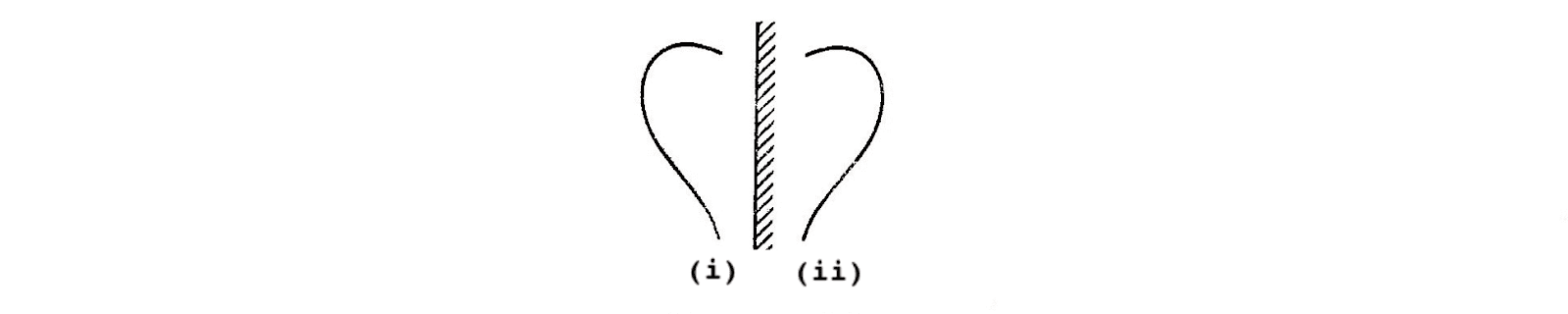In the above case, Figure (ii) is the mirror image of Figure (i). On combining these two figures we get-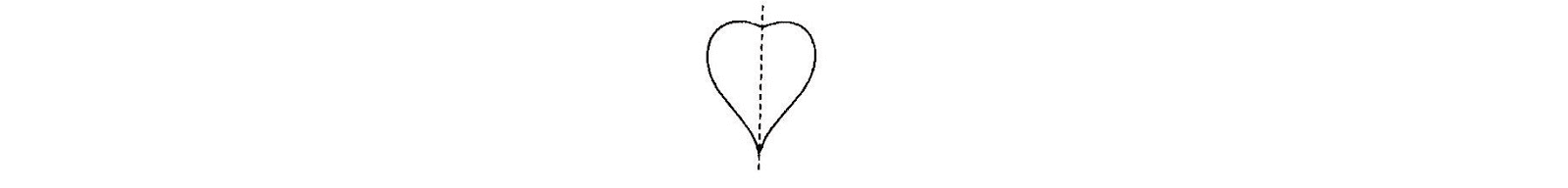We get a ‘heart’ shaped figure which is symmetrical as per the imaginary line which is drawn in place of the mirror.

### Objects having Different Mirror Images

Generally,  the mirror image of an object is different from the original object which is due to the dissimilarity between the left and right halves of the object.

Let’s look at an example to understand-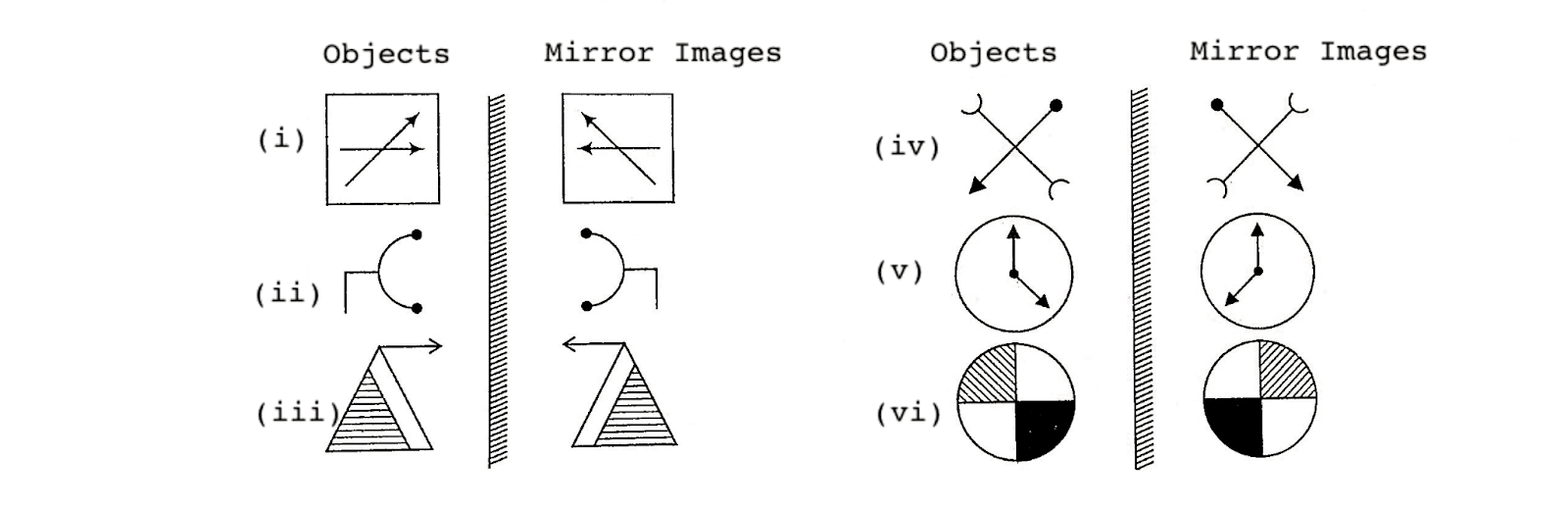From the above example it can be seen that the mirror images are different from the original object.

### Objects having Identical Mirror Images

Sometimes the mirror image formed is identical to the original object. This happens when the Left Hand Side and the Right Hand Side halves of the object are similar to each other but in opposite directions.

Let’s understand with the help of an example-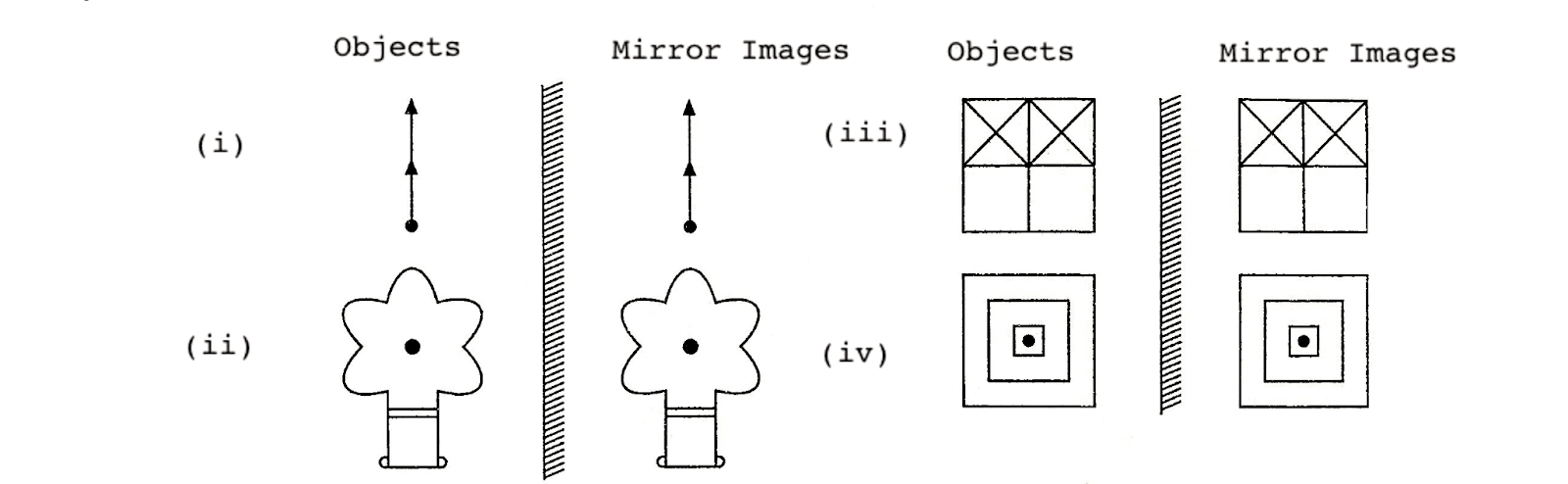In the above example, all the mirror images are identical to the original object as LHS and RHS halves of the objects are similar.

### Water Image

Water image refers to the reflection of an object into water. It is the vertically reversed image obtained by turning the object upside down. The water image of the object will look like the mirror image of the object if the mirror is placed horizontally at the bottom of the question figure.

### Objects having Different Water Images

Generally,  the water image of an object is different from the original object which is due to the dissimilarity in the upper and lower half of the object.

Let’s look at an example-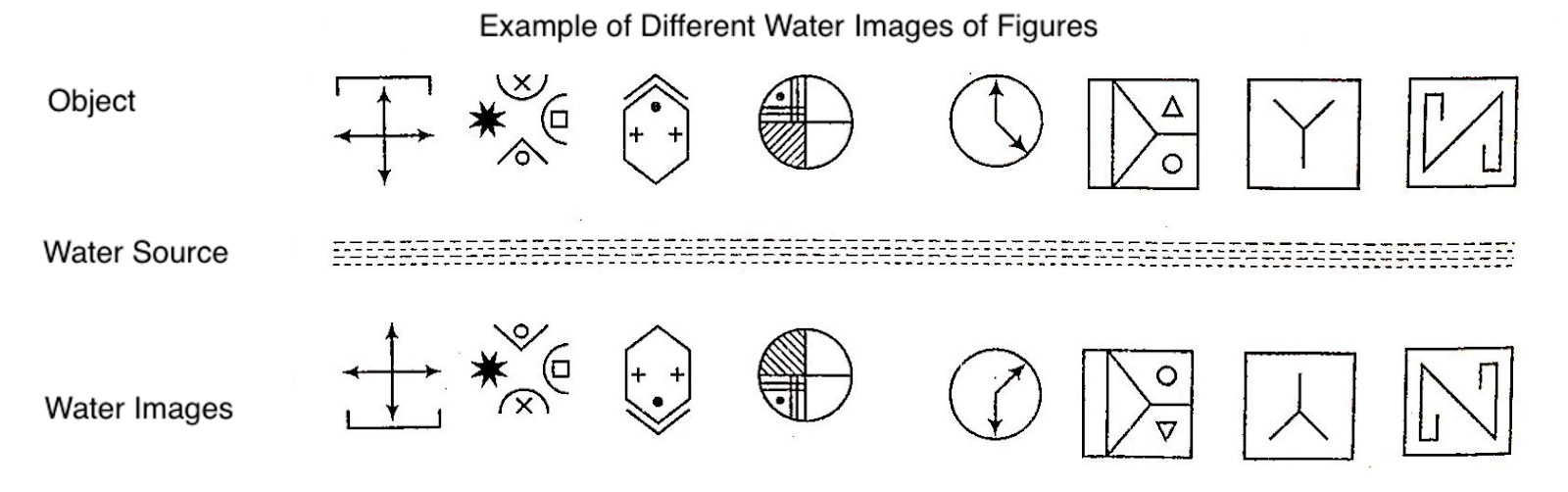In the above example, all the water images are different from the original object as there is a dissimilarity between the upper and lower halves.

### Objects having Identical Water Images

Sometimes the water image formed is identical to the original object. This happens when the upper half of the object is similar to the lower half of the object but in opposite directions.

Let’s understand with an example-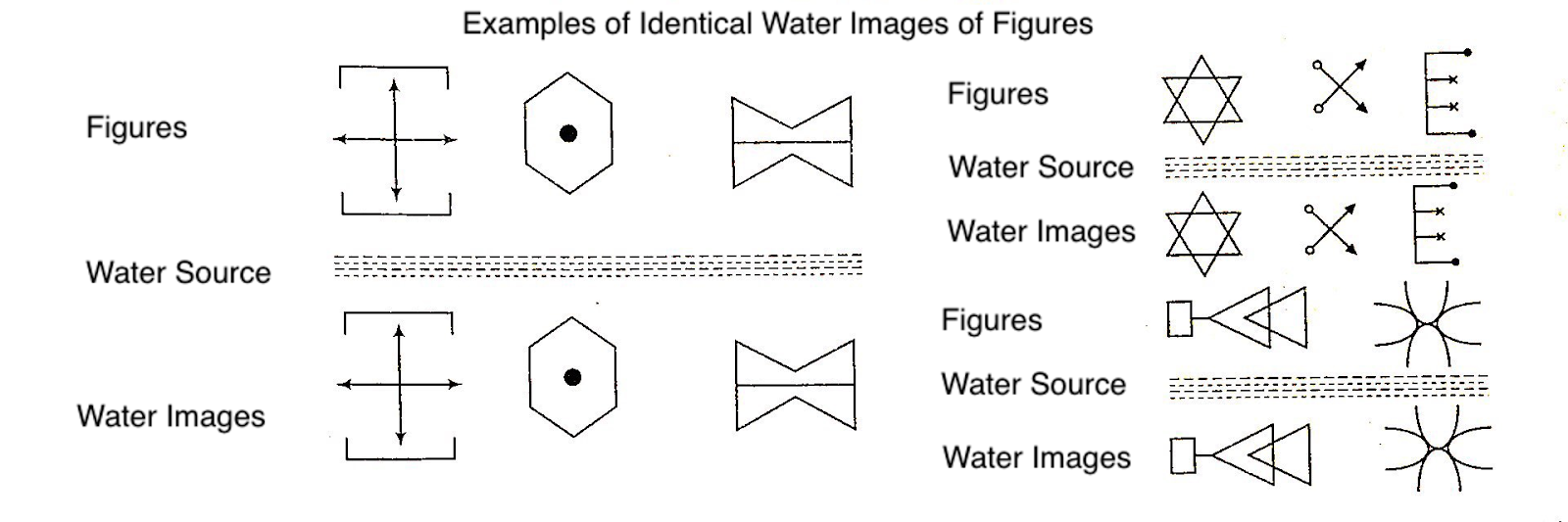In the above example, the water image is identical to the original object as the upper half of the object is similar to the lower half of the object.

From the above example, it is evident that in the water image, LHS and RHS remain the same. However, the upper and lower parts get interchanged. In other words, top becomes bottom and vice versa.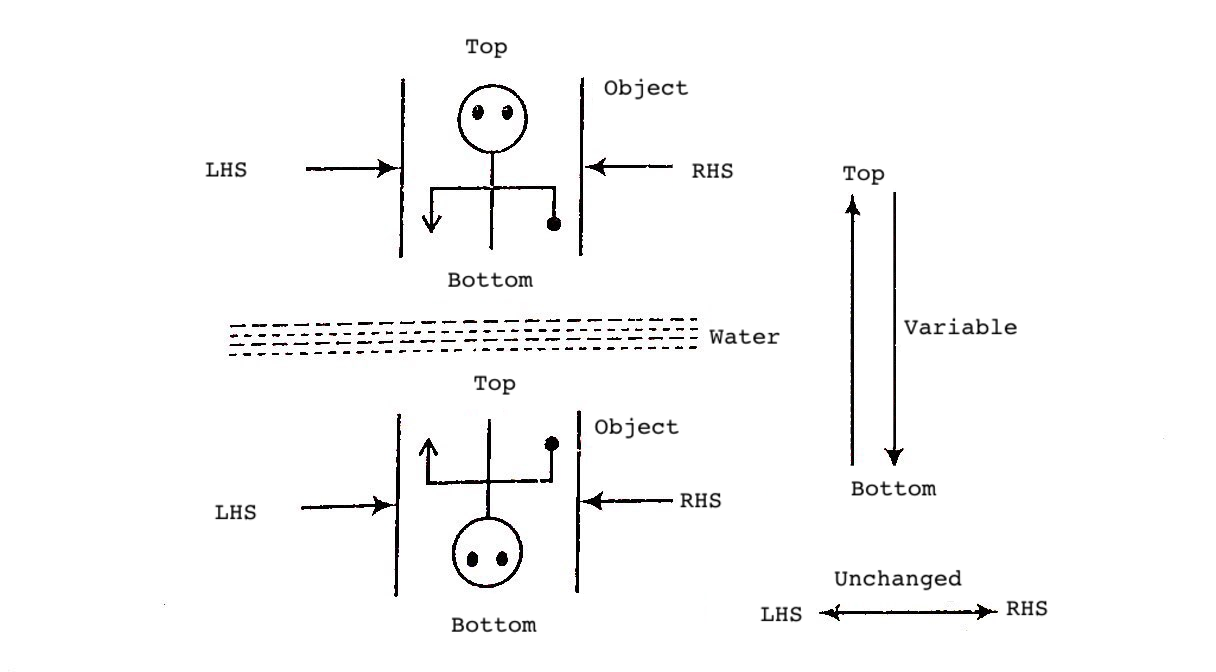## Types of Questions on Mirror Image and Water Image

Now that we have understood the basics, we look at the different types of questions that can be formed on Mirror image and Water image.

### Mirror Image

#### Based on Vertical Mirror

In these types of questions, a vertical mirror is placed on the left or right of the object and the normal form of mirror image is formed where the LHS and RHS of the object interchange their places while top and bottom remain unchanged.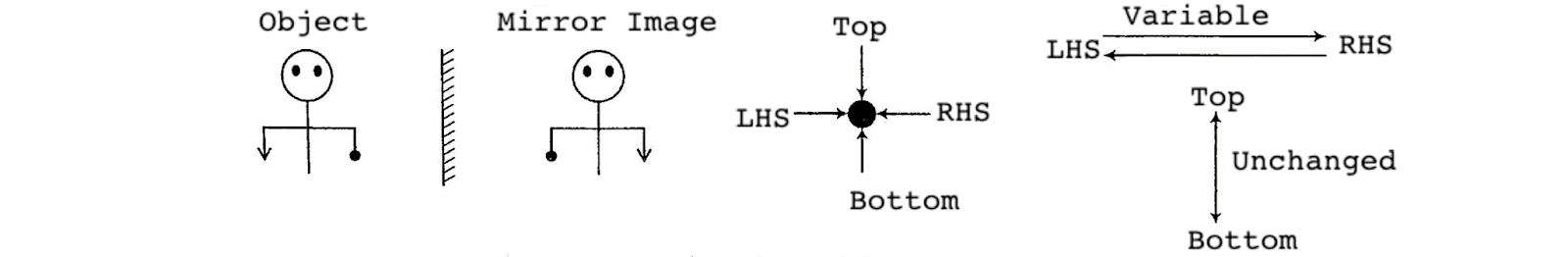To get a normal form of mirror image, the mirror is placed vertically to the left or right of the object and the image obtained in both the cases is the same.

Situation 1- When the mirror is placed vertically to the right of the object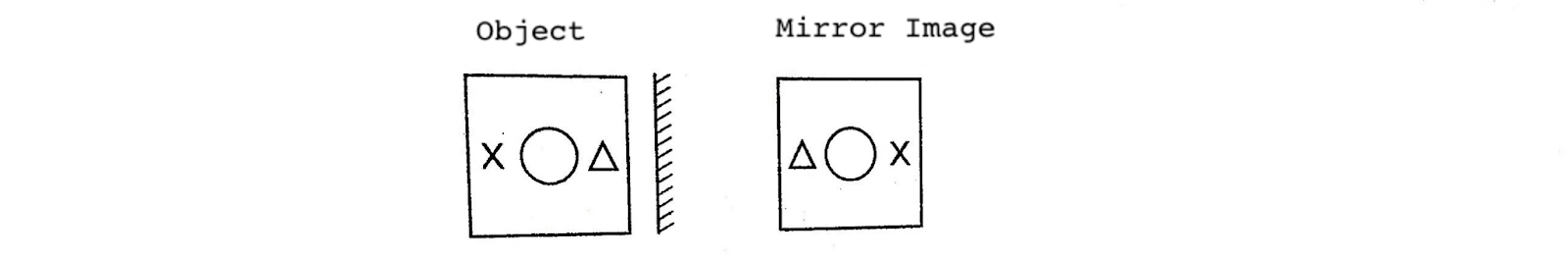Situation 2- When the mirror is placed vertically to the left of the object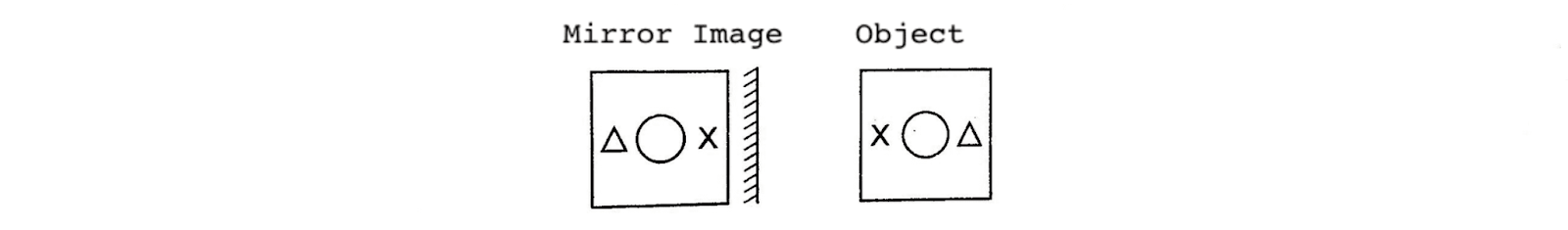Let’s look at an example-

Q1) Choose the alternatives which closely resemble the mirror image of the given combination.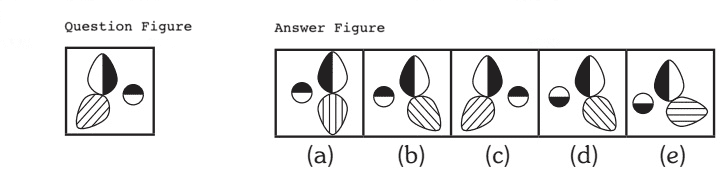Solution: (b)

Explanation:

In this example, the mirror is placed vertically on the RHS on the question figure. Hence, only the figure given in answer figure (b) would be obtained as the correct mirror image.

#### Based on Horizontal Mirror

Here, a horizontal mirror is placed at the top or bottom of the object. Therefore, the image obtained is like the water image. In such questions, RHS and LHS remain unchanged.

Situation 1- When the mirror is placed horizontally at the top of the object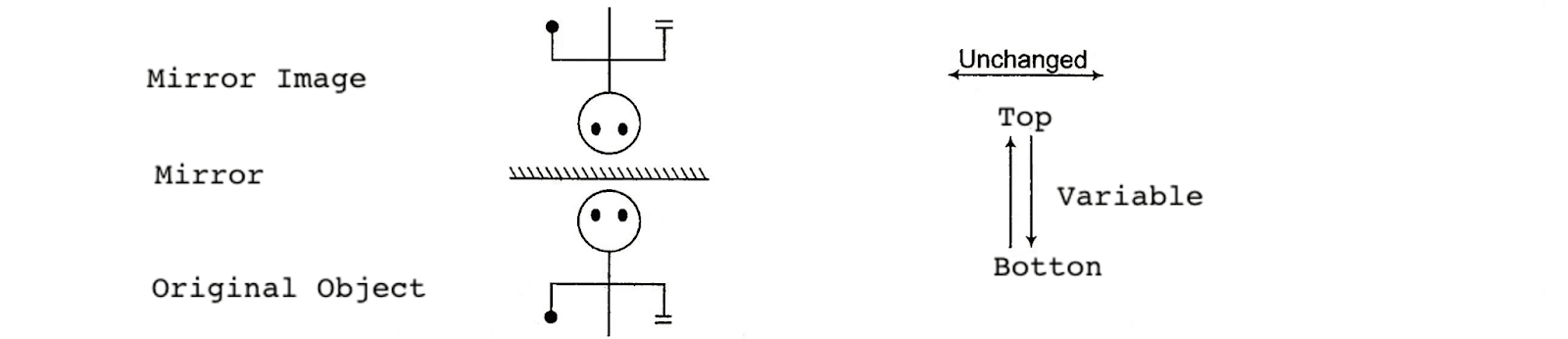Situation 2- When the mirror is placed horizontally at the bottom of the object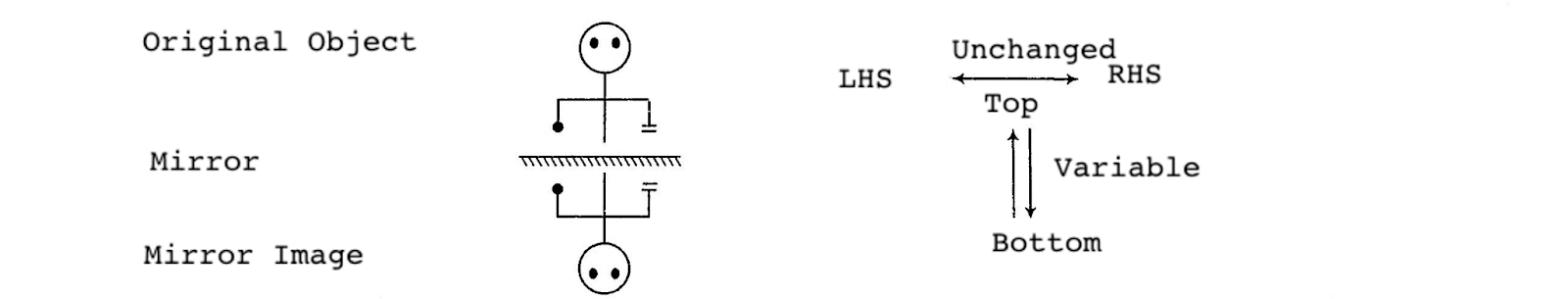Let’s understand with an example-

Q1) Choose the correct mirror image from the answer figures (a), (b), (c) and (d)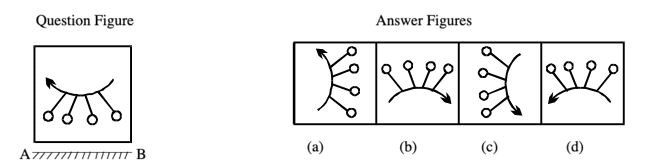Solution: (d)

Explanation:

In the example above, the mirror is placed horizontally at AB i.e., at the bottom of the question figure. Hence, only answer figure (d) looks like the water image of the question figure. It means, option (d) is the vertically inverted form of question figure in which top and bottom interchange places while LHS and RHS remain the same.

#### Mirror Image of Letters and Numbers

In these questions, a letter/group of letters and/or numbers is given and the candidates are required to determine the mirror image of the letters and numbers according to the position of the mirror placed before it.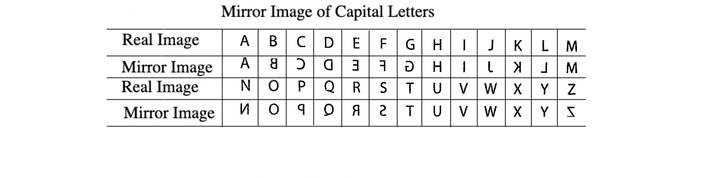Note- Letters A, H, I, M, O, T, U, V, W, X, Y have the same mirror image.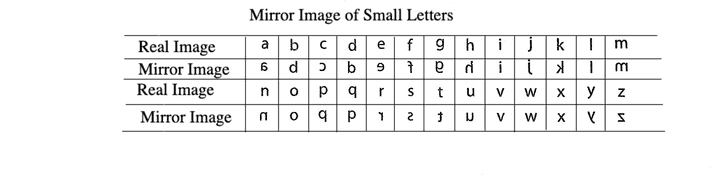Note- Letters i, l, o, v, w, x have the same mirror images.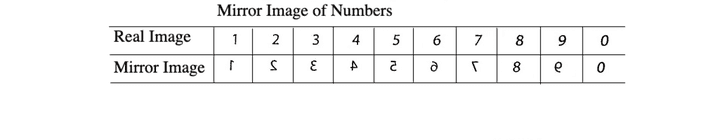Note- Numbers 0 and 8 have the same mirror image.

Let’s look at a few examples to understand-

Q1) Choose the alternative which most closely resembles the mirror image of the given combination.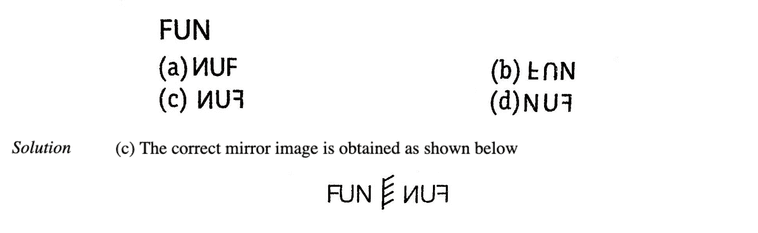Q2) Choose the alternative which most closely resembles the mirror image of the given combination.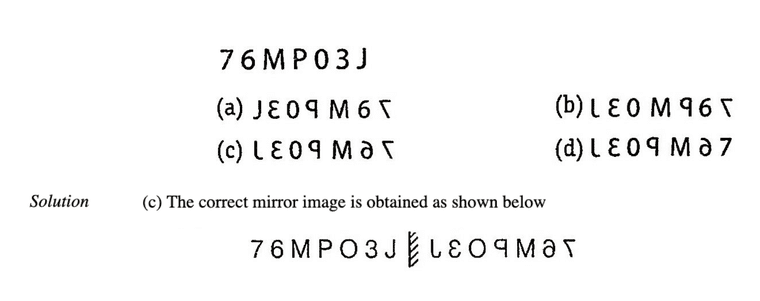#### Mirror Image of Clocks

In these types of questions, the candidates are required to find the original positions of the hour and minute hand of a clock from the positions of the hour hand and the minute hand as seen in the mirror. On the basis of the time shown by the mirror image of the clock, the candidates need to find the actual time in the clock.

For example-

Q1) A clock seen through a mirror shows quarter to three. What is the correct time shown by the clock?

(a) 8 : 15

(b) 9 : 12

(c) 8 : 17

(d) 9 : 15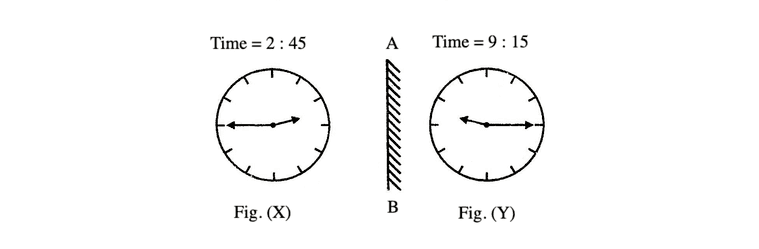Solution: (d)

Explanation:

It is evident from Figure (X), that if seen through a mirror the time indicated by clock is 2:45. When the mirror is placed vertically at AB, the mirror image i.e., Figure (Y) shows the actual time in the clock i.e., 9:15.

### Water Image

#### Water Image of Figure/Symbol/Sign

In such questions, candidates are required to find the water image of a figure/symbol/sign which consists of both symbols/signs/any other pattern.

Let’s look at an example-

Q1) Find the water image of the object given in the question figure denoted by (A), out of the figures given in the answer choices, (a), (b), (c) and (d).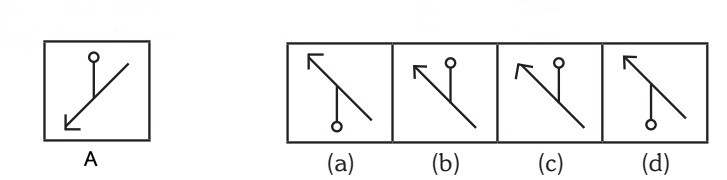Solution: (a)

Explanation:

The figure in option (a) represents the correct water image of figure (A). Upper part will go down in the opposite direction in the water image.

#### Water Image of Letters and Numbers

Here, the candidates  must know how the letters and numbers will get reflected in water and follow the same to solve the questions based on water image of letters/numbers.

Water Image of Capital Letters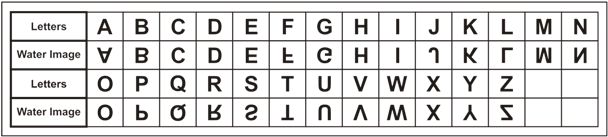Note- Letters C, D, E, H, I, O, X have identical water images.

Water Images of Small Letters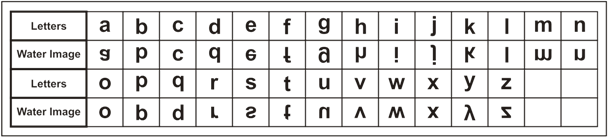Note- Letters c, i, o, x have identical water images.

Water Image of Digits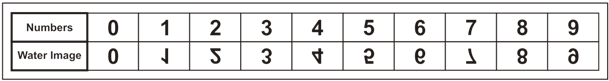Note- Numbers 0, 3 and 8 have identical water images.

Let’s look at some examples-

Q1) Find the water image of the set of letters and/or digits given in the question figure out of the four answer choices (1), (2), (3) and (4).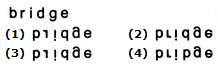Solution: (2)

Q2) Find the water image of the set of letters and/or digits given in the question figure out of the four answer choices (1), (2), (3) and (4).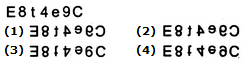Solution: (4)

## Sample Questions on Mirror Image and Water Image

Q1) Find the water image of the set of letters and/or digits given in the question figure out of the four answer choices (1), (2), (3) and (4).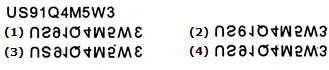Solution: (4)

Q2) Choose the alternative which closely resembles the water-image of the given combination.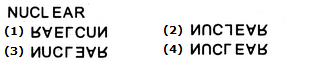Solution: (1)

Explanation:

Option (1) can be eliminated since it is laterally inverted as well. Again, in (2), L and in (3), E is laterally inverted as well. Only (4) is the correct water image.

Q3) Choose the alternative which most closely resembles the mirror image of the given combination.Q4) Identify the mirror image of the given figure if the mirror is to be placed vertically to the right of the given image.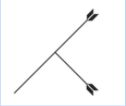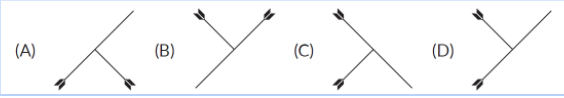Solution: (C )

Explanation:

In option A, one of the arrows facing downward in the original image is facing upward in the given option and is not correct. In option B, the original image seems to have shifted to the opposite direction and so option B is not correct. In option D, the smaller arrow seems to have shifted above the longer arrow and the longer arrow which was originally facing downward is now facing upwards and this option is also not correct. Thus, the correct answer is Option (C).

Q5) Identify the water image of the given object.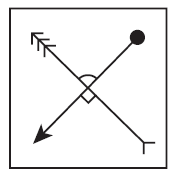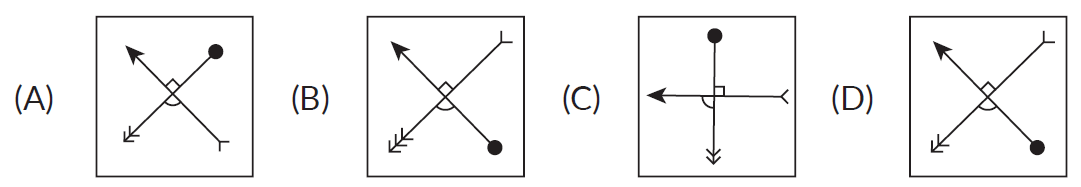Solution: (B)

Explanation:

If we see option A, the arrow is correctly facing upward but the ball is on the perpendicular arrow which is not correct. In option C, both the arrows are crossed and the ball is on the wrong arrow and so option C is not correct. In option D, one of the arrows is pointing in the correct side but the arrow which is perpendicular to this arrow is reversed and so option D is not correct. The correct option is B as it is the correct water image of the original figure.

### Conclusion

Mirror Image & Water Image is a tricky topic as it involves visualization of the question and answer figures. However, candidates can ace this topic by practicing a different variety of questions. Candidates can expect 2 to 3 questions in competitive exams.

×
×